# 利用动态规划寻找最短路径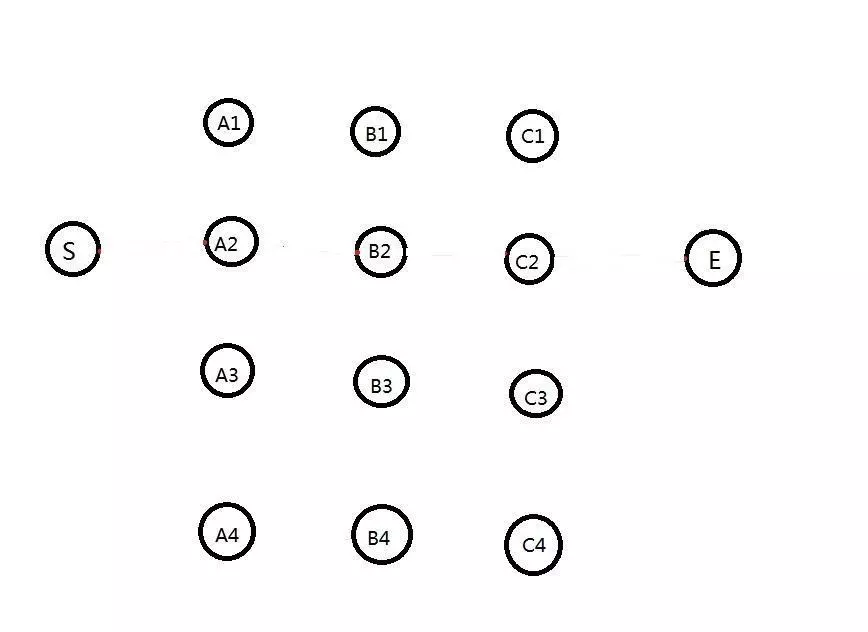## 穷举法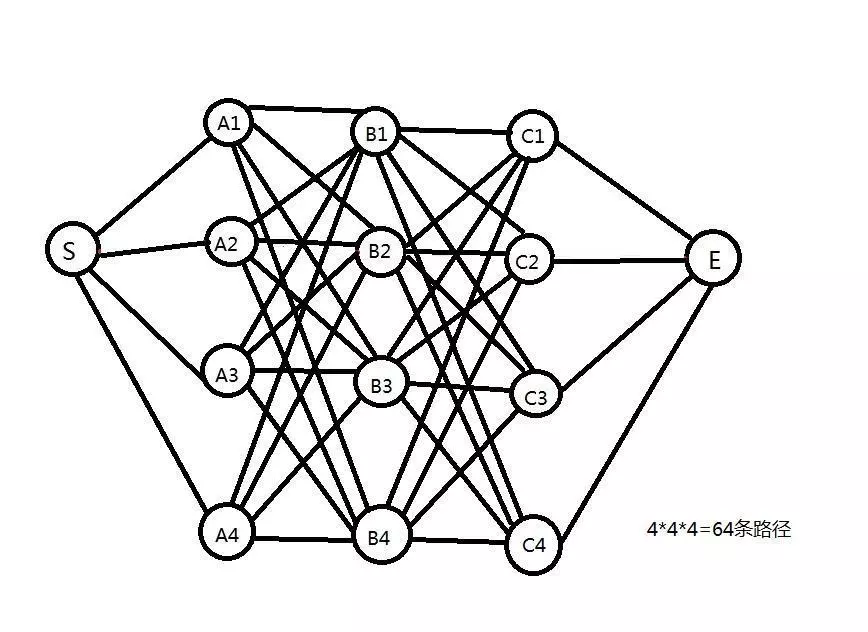## 最短路径是存在的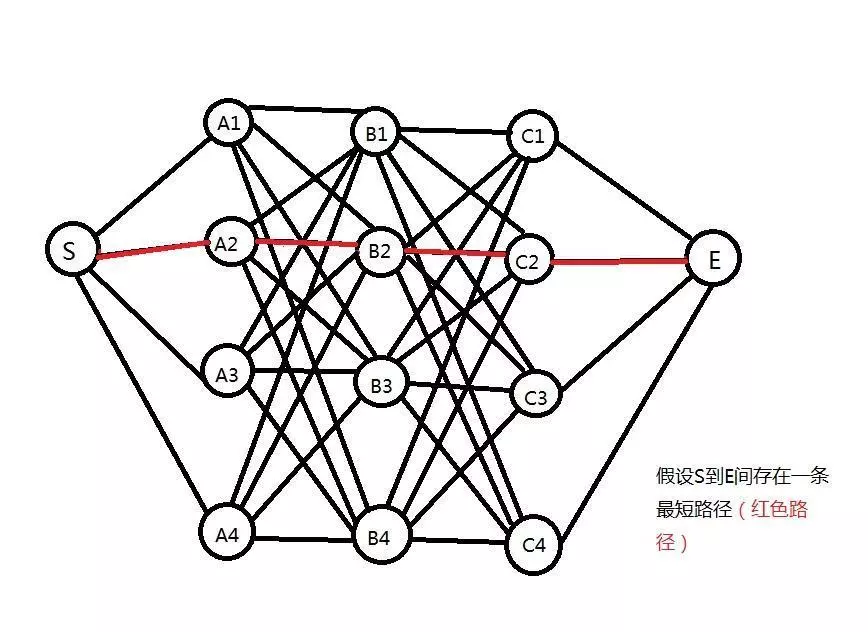（证明：反证法。如果S到C2之间存在一条更短的子路径，那么便可以用它来代替原先的路径，而原先的路径显然就不是最短了，这与原假设自相矛盾）。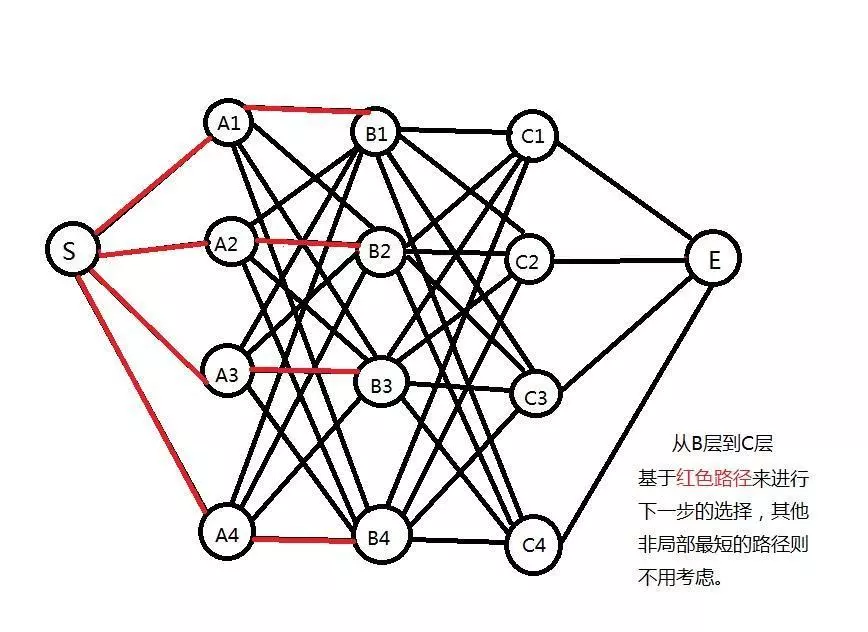OK，来到这里，我们会发现一个小“漏洞”，这段S→A2→B2→C2→E的路线只是我一厢情愿的假设，最短路径不一定是经过以上这些点。

## 完整算法

step2：对于B层的所有节点（B1,B2,B3,B4），要计算出S到它们的最短距离。我们知道，对于特定的节点B2，从S到它的路径可以经过A层的任何一个节点（A1,A2,A3,A4）。对应的路径长就是d（S,B2）=d（S,Ai）+d（Ai,B2）（其中i=1,2,3,4）。由于A层有4个节点（即i有4个取值），我们要一一计算，然后找到最小值。这样，对于B层的每个节点，都需要进行4次运算，而B层有4个节点，所以共有4X4=16次运算。

step3：这一步是该算法的核心。我们从step2计算得出的结果只保留4个最短路径值（每个节点保留一个）。那么，若从B层走向C层来说，该步骤的基数已经不再是4X4，而是变成了4！也就是说，从B层到C层的最短路径只需要基于B层得出的4个结果来计算。这种方法一直持续到最后一个状态，每一步计算的复杂度为相邻两层的计算复杂度为4X4乘积的正比！再通俗点说，连接这两两相邻层的计算符合变成了“+”号，取代了原先的“X”号。用这种方法，只需进行4X4X2=32次计算！

## 代码实现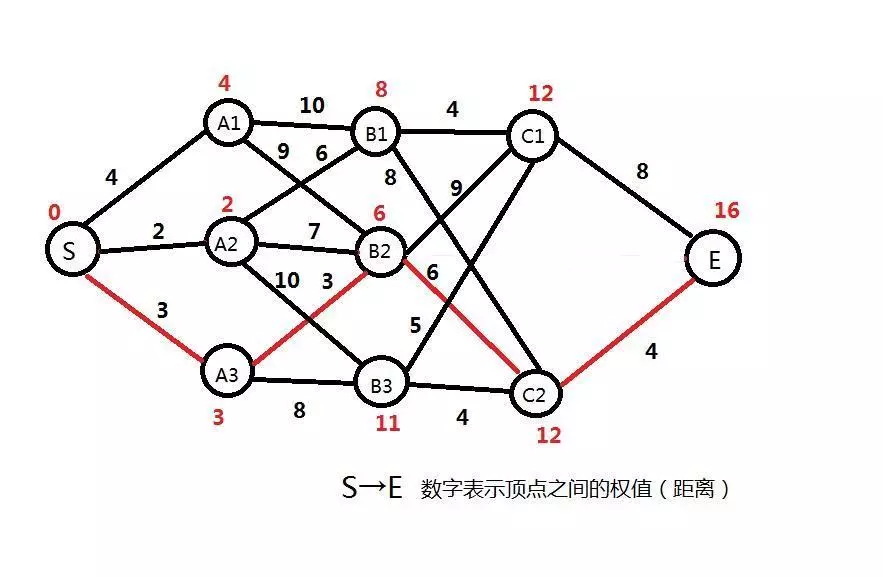#include<stdlib.h>
#include<stdio.h>
#define x 9999
#define max 9999
int data;
int dist;//记录最短路径为多少
int path;//记录最短路径
int kmin(int,int);
void fpath(int a[]);
int froute(int a[]);
void main()
{
int i,m;
int a=
{
{x,4,2,3,x,x,x,x,x,x},
{x,x,x,x,10,9,x,x,x,x},
{x,x,x,x,6,7,10,x,x,x},
{x,x,x,x,x,3,8,x,x,x},
{x,x,x,x,x,x,x,4,8,x},
{x,x,x,x,x,x,x,9,6,x},
{x,x,x,x,x,x,x,5,4,x},
{x,x,x,x,x,x,x,x,x,8},
{x,x,x,x,x,x,x,x,x,4},
{x,x,x,x,x,x,x,x,x,x}
};
/*for (i=0;i<10;i++)
{
for(j=0;j<10;j++)
printf("%d ",a[i][j]);
printf("\n");
}*/
fpath(a);
printf("最短路径大小为: %d\n",dist);

m=froute(a);
for(i=m-1;i>=0;i--)
printf("最短路径经过: %d\n",path[i]);
}
void fpath(int a[])
{
int i,j,k;42 dist=0;
for(i=1;i<10;i++)
{
k=max;
for(j=0;j<i;j++)
{
if(a[j][i]!=x)
if((dist[j]+a[j][i])<k)
k=dist[j]+a[j][i];
}
dist[i]=k;
}
}
int froute(int a[])
{
int j,b,k=1,i=9;
path=10;
while(i>0)
{
for(j=i-1;j>=0;j--)
{
if(a[j][i]!=x)
{
b=dist[i]-a[j][i];
if(b==dist[j])
{
path[k++]=j+1;
i=j;
break;
}
}
}
}
return k;
}


# 设计最短路径的动态规划算法

1）分析最优解的结构

2）递归定义最优解的值

3）自底向上计算最优解的值

4）从计算的最优解的值上面构建出最优解

## 给定一个有向图

### 有向图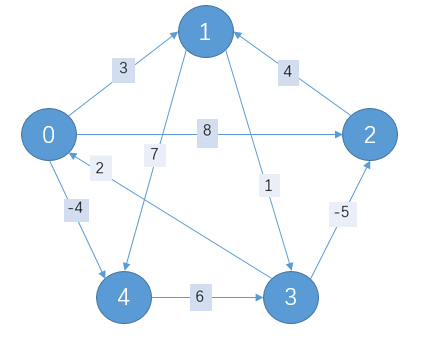### 邻接矩阵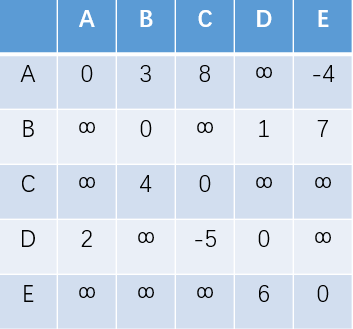# 参考资料

【算法复习】动态规划

https://www.jianshu.com/p/3b6569cefd0e